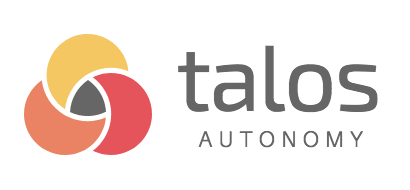## How to recover best model from experiment log?¶

Due to system error or other reason where scan_object is no longer available, it's still possible to get best model/s using nothing but the experiment log. In the below notebook you will learn exactly how.

In [ ]:
import talos
import wrangle
from tensorflow.keras.models import Sequential
from tensorflow.keras.layers import Dense


First we'll have to perform the Scan() experiment to produce the experiment log. Because the experiment log is stored on local machine, interrupted Scan() or other reason will not affect its availability. The experiment log is updated after each permutation; it contains an up-to-date record of the experiment.

In [ ]:
# load the data
x, y = talos.templates.datasets.iris()
x_train, y_train, x_val, y_val = wrangle.array_split(x, y, .3)

# set the parameter space boundary
p = {'activation':['relu', 'elu'],
'optimizer': ['Nadam', 'Adam'],
'losses': ['logcosh'],
'shapes': ['brick'],
'first_neuron': [16, 32, 64, 128],
'hidden_layers':[0, 1, 2, 3],
'dropout': [.2, .3, .4],
'batch_size': [20, 30, 40, 50],
'epochs': }

# define the input model
def iris_model(x_train, y_train, x_val, y_val, params):

model = Sequential()
model.add(Dense(params['first_neuron'], input_dim=4, activation=params['activation']))

talos.utils.hidden_layers(model, params, 3)

model.add(Dense(3, activation='softmax'))
model.compile(optimizer=params['optimizer'], loss=params['losses'], metrics=['acc'])

out = model.fit(x_train, y_train, callbacks=[talos.utils.ExperimentLogCallback('minimal_iris', params)],
batch_size=params['batch_size'],
epochs=params['epochs'],
validation_data=[x_val, y_val],
verbose=0)

return out, model

# start the experiment
scan_object = talos.Scan(x=x_train,
y=y_train,
x_val=x_val,
y_val=y_val,
model=iris_model,
experiment_name='minimal_iris',
params=p,
round_limit=10)


Now we can assume the case where we no longer have access to the scan_object. In this Scan(...experiment_name...) was set to "reactivate" so we'll find a folder with that name in the present working directory. Next we have to find out what is the name of the experiment log.

In [ ]:
# get the name of the experiment log
!ls -lhtr minimal_iris


What you want to do, is get the name of the .csv file you want to use, and use it as part of the input for experiment_log in the next step.

In [ ]:
from talos.utils.recover_best_model import recover_best_model

results, models = recover_best_model(x_train=x_train,
y_train=y_train,
x_val=x_val,
y_val=y_val,
experiment_log='minimal_iris/012620102735.csv',
input_model=iris_model,
n_models=5,
task='multi_label')


Now we can access the cross-validation results:

In [ ]:
results


We can also access the models and make predictions with them:

In [ ]:
models.predict(x_val)